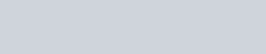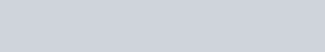# NCERT Solutions For Class 12 Chemistry Chapter 3 Electrochemistry

3.1 Arrange the following metals in the order in which they displace each other from the solution of their salts: Al, Cu, Fe, Mg and Zn.
Sol. Mg, Al, Zn, Fe, Cu, Ag.

3.2 Given the standard electrode potentials, K+/K=-2. 93 V, Ag+/Ag = 0.80 V, Hg2+/Hg =0.79V, Mg2+/Mg=-2.37V, Cr3+/Cr=0.74V.
Arrange these metals in their increasing order of reducing power.
Sol. Higher the oxidation potential more easily it is oxidized and hence greater is the reducing power. Thus, increasing order of reducing power will be Ag<Hg<Cr<Mg<K.

3.3 Depict the galvanic cell in which the reaction
Zn(s) + 2Ag+(aq) —-> 7M2+(aq) + 2Ag (s) takes place. Further show:
(i) Which of the electrode is negatively charged?
(ii) The carriers of the current in the cell.
(iii) Individual reaction at each electrode.
Sol. The set-up will be similar to as shown below,(i) Anode, i. e, zinc electrode will be negatively charged.
(ii) The current will flow from silver to copper in the external circuit.
(iii) At anode: Zn(s) ——–> Zn2+(aq) + 2e
At cathode: 2Ag+(aq) + 2e ——–> 2Ag(s)

3.4 Calculate the standard cell potentials of galvanic cell in which the following reactions take placeSol.3.5 Write the Nernst equation and emf of the following cells at 298 K:Sol.3.6 In the button cells widely used in watches and other devices the following reaction takes place:Sol.3.7 Define conductivity and molar conductivity for the solution of an electrolyte. Discuss their variation with concentration.
Sol. Conductivity of a solution is defined as the conductance of a solution of 1 cm length and having 1 sq. cm as the area of cross-section.
Molar conductivity of a solution at a dilution (V) is the conductance of all the ions produced from one mole of the electrolyte dissolved in V cm3 of the solution when the electrodes are one cm apart and the area of cross-section of the electrodes is so large that the whole of the solution is contained between them. It is usually represented by Λm.
The conductivity of a solution (both for strong and weak electrolytes) decreases with decrease in concentration of the electrolyte, i.e., on dilution. This is due to the decrease in the number of ions per unit volume of the solution on ‘ dilution. The molar conductivity of a solution increases with decrease in concentration of the electrolyte. This is because both number of ions as well as mobility of ions increases with dilution. When concentration approaches zero, the molar conductivity is known as limiting molar conductivity.

3.8 The conductivity of 0.20 M solution of KCl at 298 K is 0.0248 S cm-1. Calculate its molar conductivity.
Sol.3.9 The resistance of a conductivity cell containing 0.001 M KCI solution at 298 K is 1500 Ω What is the cell constant if conductivity of 0.001 M KCI solution at 298 K is 0.146 x 10-3 S cm-1?
Sol.3.10 The conductivity of NaCl at 298 K has been determined at different concentrations and the results are given below:Sol.3.11 Conductivity of 0.00241 M acetic acid is 7.896 x 10-5 S cm-1. Calculate its molar conductivity. If Λm0, for acetic acid is 390.5 S cm2 mol-1, what is its dissociation constant?
Sol.3.12 How much charge is required for the following reductions:
(i) 1 mol of Al3+ to Al?
(ii) 1 mol of Cu2+ to Cu ?
(iii) 1 mol of Mn04- to Mn2+?
Sol. (i) The electrode reaction is Al3+ + 3e ——> Al
∴ Quantity of charge required for reduction of 1 mol of Al3+=3F=3 x 96500C=289500C.
(ii) The electrode reaction is Cu2+ + 2e ——–> Cu
∴ Quantity of charge required for reduction of 1 mol of Cu2+=2F=2 x 96500=193000 C.
(iii) The electrode reaction is Mn04- ———-> Mn2+.
i.e., Mn7+ + 5e——-> Mn2+.
∴ Quantity of charge required = 5F
=5 x 96500 C=4825000.

3.13 How much electricity in terms of Faraday is required to produce .
(i) 20.0 g of Ca from molten CaCl2?
(ii) 40.0 g of Al from molten Al203?
Sol.3.14 How much electricity is required in coulomb for the oxidation of (i) 1 mol of H2O to 02 (ii) 1 mol of FeO to Fe203
Sol.3.15 A solution of Ni(N03)2 is electrolyzed between platinum electrodes using a current of 5 amperes for 20 minutes. What mass of Ni is deposited at the cathode?
Sol.3.16 Three electrolytic cells A, B, C containing solutions of ZnS04, AgNO3 and CuS04, respectively are connected in series. A steady current of 1.5 amperes was passed through them until 45 g of silver deposited at the cathode of call B. How long did the current flow? What mass of copper and zinc were deposited?
Sol.3.17 Predict if the reaction between the following is feasible:Sol. The reaction is feasible if the EMF of the cell reaction is positive.3.18 Predict the products of electrolysis in each of the following.
(i) An aqueous solution of AgNO3 with silver electrodes.
(ii) An aqueous solution of AgNO3 with platinum electrodes.
(iii) A dilute solution of H2S04 with platinum electrodes.
(iv) An aqueous solution of CuCl2 with platinum electrodes.
Sol.